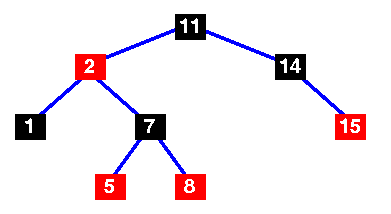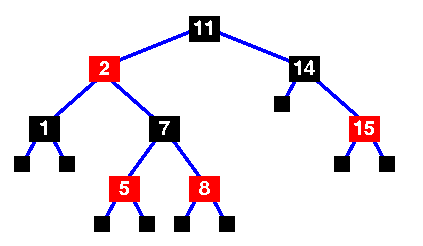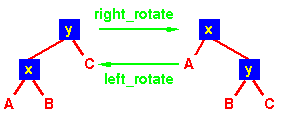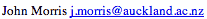Data Structures and Algorithms 8.2 Red-Black Trees

A red-black tree is a binary search tree with one extra attribute for each node: the colour, which is either red or black. We also need to keep track of the parent of each node, so that a red-black tree's node structure would be:

```struct t_red_black_node {
enum { red, black } colour;
void *item;
struct t_red_black_node *left,
*right,
*parent;
}
```
For the purpose of this discussion, the NULL nodes which terminate the tree are considered to be the leaves and are coloured black.

#### Definition of a red-black tree

A red-black tree is a binary search tree which has the following red-black properties:
 Every node is either red or black. Every leaf (NULL) is black. If a node is red, then both its children are black. Every simple path from a node to a descendant leaf contains the same number of black nodes. implies that on any path from the root to a leaf, red nodes must not be adjacent. However, any number of black nodes may appear in a sequence.A basic red-black treeBasic red-black tree with the sentinel nodes added. Implementations of the red-black tree algorithms will usually include the sentinel nodes as a convenient means of flagging that you have reached a leaf node. They are the NULL black nodes of property 2.
The number of black nodes on any path from, but not including, a node x to a leaf is called the black-height of a node, denoted bh(x). We can prove the following lemma:
##### Lemma
A red-black tree with n internal nodes has height at most 2log(n+1).
(For a proof, see Cormen, p 264)

This demonstrates why the red-black tree is a good search tree: it can always be searched in O(log n) time.

As with heaps, additions and deletions from red-black trees destroy the red-black property, so we need to restore it. To do this we need to look at some operations on red-black trees.

#### Rotations

 A rotation is a local operation in a search tree that preserves in-order traversal key ordering. Note that in both trees, an in-order traversal yields: `A x B y C`The left_rotate operation may be encoded:
```left_rotate( Tree T, node x ) {
node y;
y = x->right;
/* Turn y's left sub-tree into x's right sub-tree */
x->right = y->left;
if ( y->left != NULL )
y->left->parent = x;
/* y's new parent was x's parent */
y->parent = x->parent;
/* Set the parent to point to y instead of x */
/* First see whether we're at the root */
if ( x->parent == NULL ) T->root = y;
else
if ( x == (x->parent)->left )
/* x was on the left of its parent */
x->parent->left = y;
else
/* x must have been on the right */
x->parent->right = y;
/* Finally, put x on y's left */
y->left = x;
x->parent = y;
}
```

#### Insertion

Insertion is somewhat complex and involves a number of cases. Note that we start by inserting the new node, x, in the tree just as we would for any other binary tree, using the tree_insert function. This new node is labelled red, and possibly destroys the red-black property. The main loop moves up the tree, restoring the red-black property.
```rb_insert( Tree T, node x ) {
/* Insert in the tree in the usual way */
tree_insert( T, x );
/* Now restore the red-black property */
x->colour = red;
while ( (x != T->root) && (x->parent->colour == red) ) {
if ( x->parent == x->parent->parent->left ) {
/* If x's parent is a left, y is x's right 'uncle' */
y = x->parent->parent->right;
if ( y->colour == red ) {
/* case 1 - change the colours */
x->parent->colour = black;
y->colour = black;
x->parent->parent->colour = red;
/* Move x up the tree */
x = x->parent->parent;
}
else {
/* y is a black node */
if ( x == x->parent->right ) {
/* and x is to the right */
/* case 2 - move x up and rotate */
x = x->parent;
left_rotate( T, x );
}
/* case 3 */
x->parent->colour = black;
x->parent->parent->colour = red;
right_rotate( T, x->parent->parent );
}
}
else {
/* repeat the "if" part with right and left
exchanged */
}
}
/* Colour the root black */
T->root->colour = black;
}

```

### Animation

Red-Black Tree Animation
This animation was written by Linda Luo, Mervyn Ng, Anita Lee, John Morris and Woi Ang.©, 1998